• Call Now

1800-102-2727•

# Shapes of Orbitals: Definition of Orbitals, Nodes, Shapes of Orbitals, Practice Problems & FAQs

Are you aware of a planned city? Chandigarh, Madurai, and Jaipur are a few examples of some planned cities. What is unique about them? These planned cities are meticulously planned and developed in infrastructure comprising of roads, houses, greenery, industries and so on.. India is known for its Town planning and architecture even before independence as existing in Madurai, a city in Tamil Nadu. But they are only a few.Coming to the smallest particles on earth, the atom, we can say, that it is also a well-planned and organized of its constituents. You know that of the three fundamental particles proton and neutron are said to form the core around which the third particle electrons are distributed uniformly.

The notion of electrons as an electron cloud of a particle mass was developed mathematically by Schrodinger. Nevertheless, the distribution of several electrons present in the atom has a specific volume for them called orbitals.

We shall know about the architectural design of electrons around the core nucleus of an atom here.

## Definition of Orbitals

The volume around the nucleus where an electron possing a given energy can be found most probably is called an orbital. The probability may or may not uniformly distributed around the nucleus and hence the shape of the region decides the shape of the orbital. The probability is given by the plot of square of angular wave functions around an origin or centre.

Since electrons are thought of as matter waves, they can be visualised as clouds as opposed to point masses. The density of electrons in an electron cloud is not uniform. Depending on how far the nucleus is, the electron density changes. The orbital is governed by the three quantum numbers, namely principal, azimuthal and magnetic quantum numbers.

Only two electrons can be accommodated in each orbital to the maximum.Orbitals also contain phases or signs that stand in for various aspects of the orbits. With distinct coloured or shaded lobes, orbital drawings are used to depict the phases, but they actually represent mathematical functions.There are four different shapes of orbitals in the naturally existing atoms, each with a unique shape and designated by the letters s (Sharp), p (Principal), d (diffuse), and f (fundamental).

Two more orbitals shapes designated as g and h can describe the electron cloud shape of synthetic atoms.

## Nodes

The probability of discovering electron density is lowest at nodes. The nodal plane, which goes through the nucleus, is the only plane where electrons are not present.

Nodes has been classified into two categories:

• Angular nodesThe electrons of different orbitals shall have the least probability in certain directions or along the radius of the atomic sphere. Accordingly, we may call them Angular nodes or radial nodes.

The number of angular nodes of an orbital is equal to the azimuthal quantum number of the orbital.

Number of angular nodes of an orbital =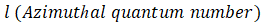Number of Radial nodes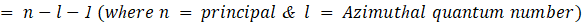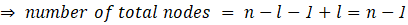Calculation of Number of Nodes:

## Orbitals and Quantum Numbers

The most electrons possible in a shell =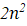Number of orbitals present in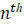shell =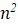Angular Momentum of any orbit =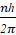Number of orbitals in a subshell =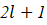Maximum number of electrons in particular subshell =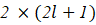Orbital Angular Momentum =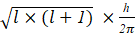Note:## Shapes of Orbitals

The Schrodinger wave equation solutions are used to plot the shape of the orbitals.

s-Orbital:

• For s-orbital l amd m quantum numbers are zero, indicating that t'he size of an s-orbital depends on the value of the primary quantum number ‘n’ only. As "n" grows, the orbital's size grows as uniformly around nucleus such that the probability of finding an electron at a given distance is same in all direction's from the nucleus is the same. Consequently, it ought to be spherical in shape. As a result, all s-orbitals are non-directional and spherically symmetric with respect to the nucleus.
• The existence of a spherical shell with a probability of finding an electron of zero in the 2s-orbital. Nodes or nodal surfaces are terms used to describe it. In the 2s orbit, there is only one spherical node. The number of nodal surfaces or nodes in the s-orbital of any energy level is exactly (n-1) where n is the primary quantum number.p-Orbital:

• For p-subshell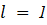, "m" can take one of three possible values: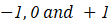. It implies that there are three possible orientations for p-orbitals. Despite having different orientations, all three of these p-orbitals have the same energy in a degenerate state. Two lobes on each p-orbital are symmetrical about a particular axis. The lobes are designated as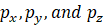depending on their orientation because they are symmetric about the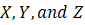axes, respectively.
• In the figure, the lines represent a cross-section of the p-three-dimensional orbital's boundary surface. The 90% of the dots that represent electrons are on a surface known as the boundary surface. A nodal plane divides each p-two orbital's lobes. For a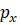orbit, the YZ plane serves as the nodal plane x.
• Thus, p-orbitals have a dumbbell-like shape and directional characteristics. The probability that the electron will be present in both lobes is equal. Even though they contain more energy, the p-orbitals of higher energy levels have similar shapes.d-Orbital:

d-subshells have l = 2, for which "m," can have five orienatations of -2, -1, 0, 1, and 2. As a result, there are five possible orientations for d-orbitals: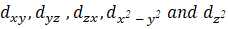. The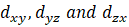orbitals all have the same shape but they are located in the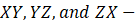planes, respectively.

The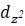orbital is symmetric about the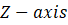and has a dumbbell-shaped electron cloud in the centre. The d-subshell has a maximum capacity of ten electrons.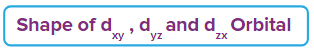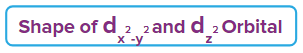## Practice Problems

Q1. What is the number of angular nodes present in 3s orbital

a. 0b. 1c. 2d. 3

3s-orbital contains a spherical shell where there is no chance of finding an electron. Nodes or nodal surfaces are terms used to describe it. In the 3s orbit, there is only one spherical node. The number of nodal surfaces or nodes in the s-orbital of any energy level is exactly (n-1) where n is the fundamental quantum number. It contains radial nodes. Hence, option A is the correct answer.

Q2. The number of radial nodes of 2s, 3p, and 4d electrons is, respectively,

a. 0, 0, 2b. 1, 1, 1c. 2, 2, 2d. 0, 0, 0

Q3. The probability of finding an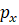electron is zero in

a. XY-planeb. YZ-planec. XZ-planed. Z-axis

The probability of not finding anelectron is zero in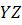plane.

Q4. Choose the correct statement.

a. p - orbital can have only 2 electrons.b. s - orbital is the most symmetric orbital.c. d -orbital is the most symmetric orbital.d. None of the above

There is only one possible orientation when l = 0, as indicated by the fact that the value of m for s-orbitals is 0. This shows that the probability of finding an electron at a given every direction's distance from the nucleus is the same. Therefore, it should have a spherical shape. Because of this, all s-orbitals are spherically symmetrical.## Frequently Asked Questions - FAQs

Q1. What do the various subshells denote?Answer: The subshells denoted with letters s, p, d, and f representing sharp, primary, diffuse, and fundamental, respectively, the notations assigned to groups of lines observed in the spectra of alkali metals.

Q2. What distinguishes an orbital from a shell/orbit?Answer:

Q3. How many subshells are there in the periodic table's elements?Answer:We only typically use the s, p, d, and f subshells to describe theelectronic configuration of the 118 elements in our periodic table. We can use subshells with g (9 orbitals), h (11 orbitals), etc. for fictitious elements.

Q4. What do you understand by the Schrodinger wave equation?Answer: The Schrodinger wave equation is a mathematical expression that accounts for the electron's nature as a matter wave inside an atom when describing the energy and position of the electron in space and time. It is base on three factors.

• Classical plane wave equation,
• Broglie’s Hypothesis of matter-wave, and
• Conservation of Energy

The form of the wave functions or probability waves that govern the motion of some smaller particles is precisely described by the Schrodinger equation. The equation also explains how various external factors impact these waves. Additionally, the equation makes use of the energy conservation principle, which provides information about how an electron attached to a nucleus behaves.

Related Topics

Talk to our expert
Resend OTP Timer =
By submitting up, I agree to receive all the Whatsapp communication on my registered number and Aakash terms and conditions and privacy policy